### IMO Shortlist 1964 problem 5

Kvaliteta:
Avg: 0.0
Težina:
Avg: 0.0
In tetrahedron$ABCD$, vertex$D$ is connected with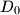$D_0$, the centrod if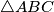$\triangle ABC$. Line parallel to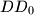$DD_0$ are drawn through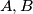$A,B$ and$C$. These lines intersect the planes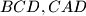$BCD, CAD$ and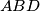$ABD$ in points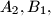$A_2, B_1,$ and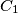$C_1$, respectively. Prove that the volume of$ABCD$ is one third the volume of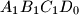$A_1B_1C_1D_0$. Is the result if point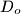$D_o$ is selected anywhere within$\triangle ABC$?
Source: Međunarodna matematička olimpijada, shortlist 1964